# Cohn's irreducibility criterion

Supposeis a polynomial with integer coefficients, i.e.,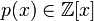. Suppose that all the coefficients ofare nonnegative. Further, suppose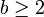is a natural number strictly greater than all coefficients. Then, if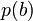is a prime number,must be an irreducible polynomial.
An alternate formulation is as follows: for any, if a number with digits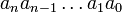written in baseis prime (so in particularfor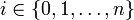) then the polynomial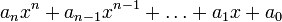is irreducible.